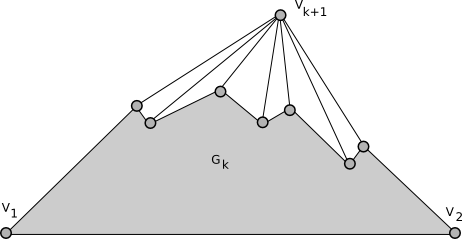#Boost C++ Libraries

...one of the most highly regarded and expertly designed C++ library projects in the world.

# Planar Canonical Ordering

```template <typename Graph, typename PlanarEmbedding, typename OutputIterator, typename VertexIndexMap>
void planar_canonical_ordering(const Graph& g, PlanarEmbedding embedding, OutputIterator ordering, VertexIndexMap vm);
```

A planar canonical ordering is an ordering v1, v2, ..., vn of the vertices of a maximal planar graph having the property that, for each k, 3 <= k < n, the graph induced by v1, v2, ..., vk

• is biconnected and contains the edge {v1, v2} on its outer face.
• has any vertices in the range v1, v2, ..., vk that are adjacent to v(k+1) on its outer face, and these vertices form a path along the outer face.
Let Gk be the graph induced by the first k vertices in the canonical ordering, along with all edges between any of the first k vertices. After Gk has been drawn, the (k+1)st vertex can be drawn easily without edge crossings, since it's adjacent only to a consecutive sequence of vertices on the outer face of Gk.A planar canonical ordering exists for every maximal planar graph with at least 2 vertices. planar_canonical_ordering expects the input graph to have at least 2 vertices.

The planar canonical ordering is used as an input in some planar graph drawing algorithms, particularly those that create a straight line embedding. de Fraysseix, Pach, and Pollack  first proved the existence of such an ordering and showed how to compute one in time O(n) on a maximal planar graph with n vertices.

### Complexity

If the vertex index map provides constant-time access to indices, this function takes time O(n + m) for a planar graph with n vertices and m edges. Note that in a simple planar graph with f faces, m edges, and n vertices, both f and m are O(n).

### Parameters

IN: Graph& g
An undirected graph. The graph type must be a model of VertexAndEdgeListGraph. The graph must:
• Be maximal planar.
• Have at least two vertices.
IN: PlanarEmbedding
A model of PlanarEmbedding.
IN: OutputIterator
An OutputIterator with value_type equal to graph_traits<Graph>::vertex_descriptor. The canonical ordering will be written to this iterator.
IN: VertexIndexMap vm
A Readable Property Map that maps vertices from g to distinct integers in the range [0, num_vertices(g) )
Default: get(vertex_index,g)